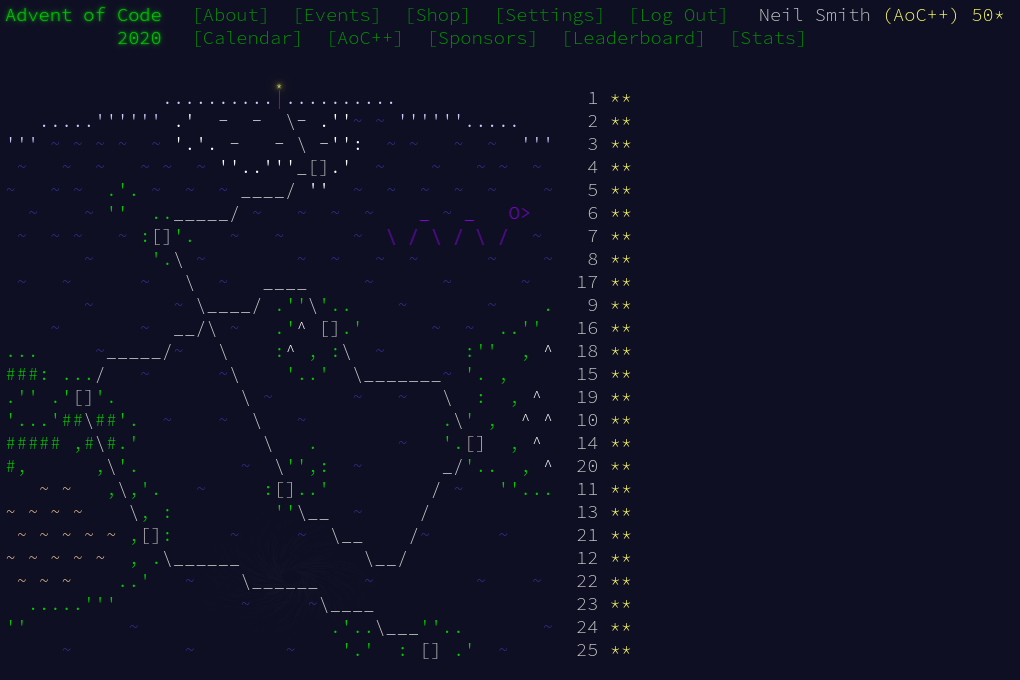January 10, 2021

# Advent of Code 2020 day 25

Avoiding the very deep weeds of number theoryDay 25 was a foray into public key encryption, using Diffie-Hellman key exchange (or should that be Ellis-Cocks-Williamson key exchange?). The idea is one of raising numbers to a power (the power being the key) and exchanging the results.

The idea is that we agree a subject number (7 in the example) and both secretly choose a key. In the example, the card's key is 8 and the door's key is 11. The card calculates 78 = 5764801 and publishes the answer. The door calculates 711 = 1977326743 and publishes that answer. The card can then calculate the encryption key as 19773267438 = (711)8 = 711 + 8. Similarly, the door calculates the encryption key as  576480111 = (78)11 = 78 + 11. These are the same numbers.

That isn't too secure, as any eavesdropper can find the keys by taking logarithms of the published numbers: $\log_7 5764801 = 8$. The complication comes from the use of modular arithmetic, where the published numbers are taken modulus some large prime. That extra layer makes the logarithms very hard to find.

Luckily, this puzzle only uses small keys, so brute-force solutions are solvable.

I started by looking at the discrete logarithm function in the arithmoi library, but got scared off by the higher-level type signature. But I did get inspired by a Reddit post, of using a newtype for numbers and defining a Semigroup using modular multiplication based on that newtype.

import Data.Semigroup

newtype MExp = MExp Integer
deriving (Show, Eq)

instance Semigroup MExp where
(MExp a) <> (MExp b) = MExp $(a * b) mod modularBase  Finding one of the keys was just counting up from 1, multiplying an accumulator until I found the target. I could have used a combination of length$ takeWhile $iterate to do that, or even an unfold, but I decided to use until again. findRounds :: Integer -> Integer findRounds target = fst$ until (foundRounds t) countingExponential (0, seed)
where t = MExp target
seed = MExp 1

foundRounds :: MExp -> (Integer, MExp) -> Bool
foundRounds target (_n, v) = v == target

countingExponential :: (Integer, MExp) -> (Integer, MExp)
countingExponential (n, v) = (n + 1, v <> subject)


All that's left is to find the encryption key, and stimes does that efficiently.

part1 cardKey doorKey = publicKey
where cardRounds = findRounds cardKey
MExp publicKey = stimes cardRounds (MExp doorKey)


Done!

And that gives me 300 stars for the six events of Advent of Code.

## Code

You can find the code here or on GitLab.